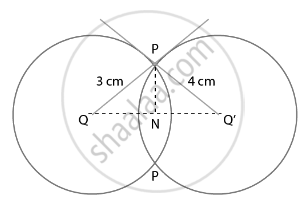# Two circles with centres O and O' of radii 3 cm and 4 cm, respectively intersect at two points P and Q such that OP and O'P are tangents to the two circles. Find the length of the common chord PQ. - Mathematics

Sum

Two circles with centres O and O' of radii 3 cm and 4 cm, respectively intersect at two points P and Q such that OP and O'P are tangents to the two circles. Find the length of the common chord PQ.

#### SolutionTwo circles with centers O and O’ of radii 3 cm and 4 cm, respectively intersect at two points P and Q, such that OP and O’P are tangents to the two circles and PQ is a common chord.

To Find: Length of common chord PQ

∠OPO’ = 90°  ......[Tangent at a point on the circle is perpendicular to the radius through point of contact]

So OPO is a right-angled triangle at P

Using Pythagoras in ΔOPO’, we have

(OO’)2= (O’P)2+ (OP)2

(OO’)2 = (4)2 + (3)2

(OO’)2 = 25

OO’ = 5 cm

Let ON = x cm and NO’ = 5 – x cm

In right angled triangle ONP

(ON)2+ (PN)2= (OP)2

x2 + (PN)2 = (3)2

(PN)2= 9 – x2   ......

In right angled triangle O’NP

(O’N)2 + (PN)2= (O’P)2

(5 – x)2 + (PN)2 = (4)2

25 – 10x + x2 + (PN)2 = 16

(PN)2 = – x2+ 10x – 9  ......

From  and 

9 – x2 = – x2 + 10x – 9

10x = 18

x = 1.8

From (1) we have

(PN)2 = 9 – (1.8)2

=9 – 3.24 = 5.76

PN = 2.4 cm

PQ = 2PN = 2(2.4) = 4.8 cm

Concept: Concept of Circle - Centre, Radius, Diameter, Arc, Sector, Chord, Segment, Semicircle, Circumference, Interior and Exterior, Concentric Circles
Is there an error in this question or solution?
Chapter 9: Circles - Exercise 9.4 [Page 110]

#### APPEARS IN

NCERT Mathematics Exemplar Class 10
Chapter 9 Circles
Exercise 9.4 | Q 5 | Page 110
Share